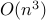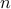# Ellipsoidal bounds for uncertain linear equations and dynamical systems

• Authors: G. Calafiore and L. El Ghaoui.

• Status: Automatica, 40 (2004) 773–787.

• Abstract: In this paper, we discuss semidefinite relaxation techniques for computing minimal size ellipsoids that bound the solution set of a system of uncertain linear equations. The proposed technique is based on the combination of a quadratic embedding of the uncertainty, and the S-procedure. This formulation leads to convex optimization problems that can be essentially solved in,being the size of unknown vector, by means of suitable interior point barrier methods, as well as to closed form results in some particular cases. We further show that the uncertain linear equations paradigm can be directly applied to various state-bounding problems for dynamical systems sub ject to set-valued noise and model uncertainty.

• Related entries:

• Bibtex reference:

```@article{CaE:04,
Author = {G. Calafiore and L. {El Ghaoui}},
Journal = {Automatica},
Pages = {773--787},
Title = {Ellipsoidal bounds for uncertain linear equations and dynamical systems},
Volume = {40},
Year = {2004}}
```